Homework Help Question & Answers

# equation 4 = MNaOH = mKHP/(MWKHP(V2 ? V1)NaOH) Write the equation and determine all the partial...

equation 4 = MNaOH = mKHP/(MWKHP(V2 ? V1)NaOH) Write the equation and determine all the partial derivatives that are used to calculate the uncertainty (error) in the NaOH concentration? Be sure to use eq(4). the question is number 8 on the pictures!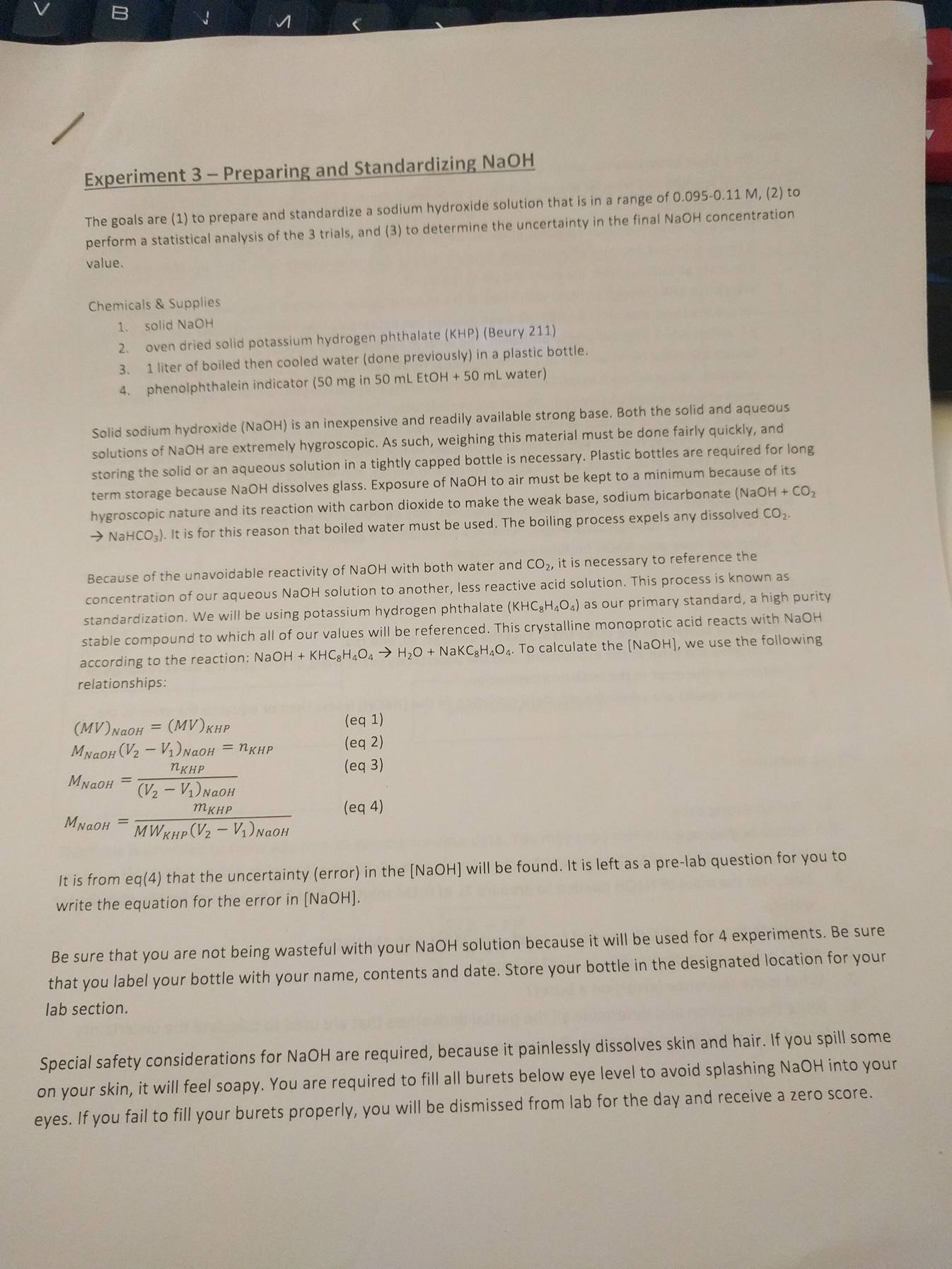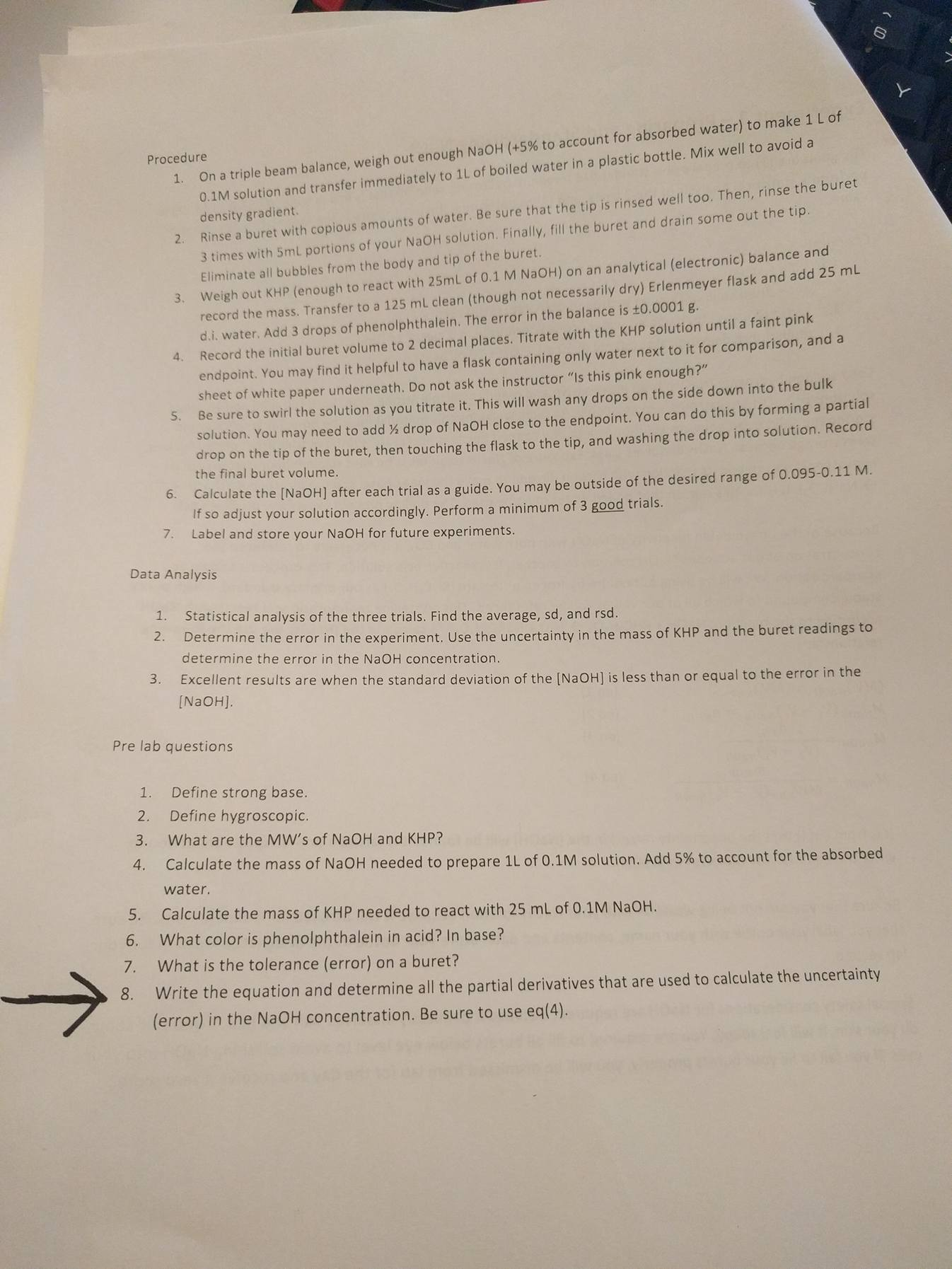Numer 8 please, I dont need any of the other questions

Experiment 3 -Preparing and Standardizing NaOH The goals are (1) to prepare and standardize a sodium hydroxide solution that is in a range of 0.095-0.11 M, (2) to perform a statistical analysis of the 3 trials, and (3) to determine the uncertainty in the final NaOH concentration value. Chemicals & Supplies 1. 2. 3. 4. solid NaOH oven dried solid potassium hydrogen phthalate (KHP) (Beury 211) 1 liter of boiled then cooled water (done previously) in a plastic bottle phenolphthalein indicator (50 mg in 50 mL EtOH+50 mL water) Solid sodium hydroxide (NaOH) is an inexpensive and readily available strong base. Both the solid and aqueous solutions of NaOH are extremely hygroscopic. As such, weighing this material must be done fairly quickly, and term storage because NaOH dissolves glass. Exposure of NaOH to air must be kept to a minimum because of its → NaHCO3). It is for this reason that boiled water must be used. The boiling process expels any dissolved CO2 storing the solid or an aqueous solution in a tightly capped bottle is necessary. Plastic bottles are required for long hygroscopic nature and its reaction with carbon dioxide to make the weak base, sodium bicarbonate (NaOH + CO2 Because of the unavoidable reactivity of NaOH with both water and CO, it is necessary to reference the concentration of our aqueous NaOH solution to another, less reactive acid solution. This process is known as standardization. We will be using potassium hydrogen phthalate (KHC3M,0s) as our primary standard, a high purity stable compound to which all of our values will be referenced. This crystalline monoprotic acid reacts with NaOH according to the reaction: NaOH + KHC8H404 → H2O + Na KC8H,04. To calculate the [NaOH), we use the following relationships (eq 1) (eq 2) (eq 3) (MV)NaOH = (MV)KHP MyaOH(V2-V1) NaOH = nKHP KHP MN mKHP (eq 4) NaOH It It is from eq(4) that the uncertainty (error) in the [NaOH] will be found. It is left as a pre-lab question for you to write the equation for the error in (NaOH] Be sure that you are not being wasteful with your NaOH solution because it will be used for 4 experiments. Be sure that you label your bottle with your name, contents and date. Store your bottle in the designated location for your lab section. Special safety considerations for NaOH are required, because it painlessly dissolves skin and hair. If you spill some on your skin, it will feel soapy. You are required to fill all burets below eye level to avoid splashing NaOH into your eyes. If you fail to fill your burets properly, you will be dismissed from lab for the day and receive a zero score.

#### Homework Answers

Answer #1

Equation 4 is

MNaOH = mKHP / MWKHP (V2-V1)NaOH

so concentration of NaOH is a function of mass of KHP, mol wt of KHP and volume of NaOH

d(MNaOH) = f(d(mKHP), d(MWKHP), d((V2-V1)NaOH))

The total deviation of concentration can be calculated by taking partial derivatives of x with respect to each term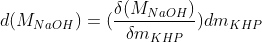,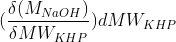,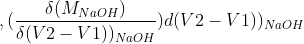Now on squaring and crossing the terms and dividing by N-1 we will get the equation for propagation of error.

σ2(MNaOH )=(δMNaOH / δmKHP)2σ2mKHP + (δMNaOH / δMWKHP)2σ2MWKHP + (δMNaOH / δ(V2-V1)NaOH)2σ2V2-V1)NaOH

Know the answer?
Your Answer:

#### Post as a guest

Your Name:

What's your source?

#### Earn Coin

Coins can be redeemed for fabulous gifts.

Not the answer you're looking for? Ask your own homework help question. Our experts will answer your question WITHIN MINUTES for Free.
Similar Homework Help Questions
• ### CHEM LAB hello can someone help me come up with a procedure for this lab it...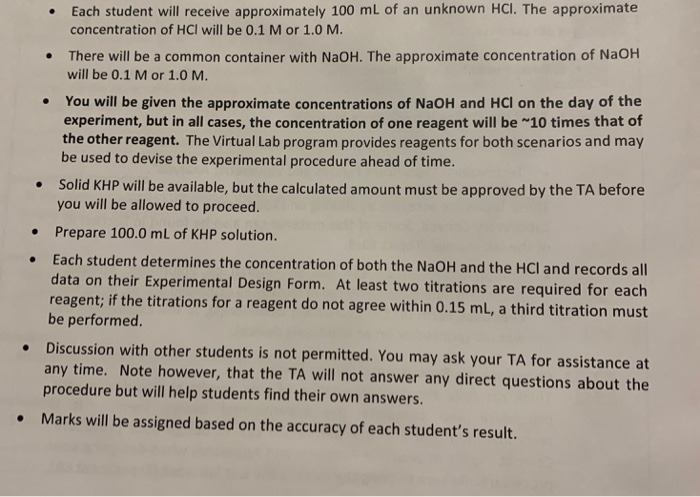CHEM LAB hello can someone help me come up with a procedure for this lab it is an standarization of acid and base solution • Each student will receive approximately 100 mL of an unknown HCl. The approximate concentration of HCl will be 0.1 M or 1.0 M. There will be a common container with NaOH. The approximate concentration of NaOH will be 0.1 M or 1.0 M. You will be given the approximate concentrations of NaOH and HCl on...

• ### not sure if molarity of NaOH was calculated correctly and also unsure of how to find...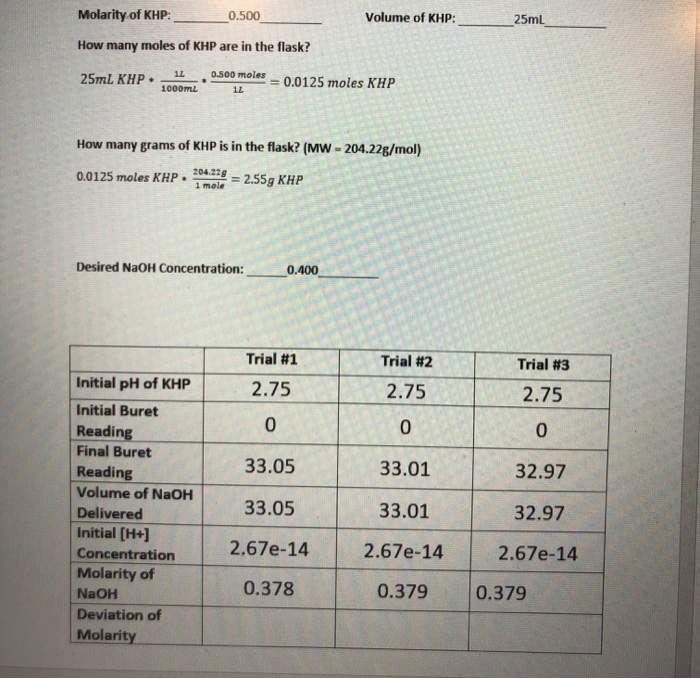not sure if molarity of NaOH was calculated correctly and also unsure of how to find deviation Molarity of KHP: _0.500 Volume of KHP: 25mL How many moles of KHP are in the flask? 1L 0.500 moles - 0.0125 moles KHP 25mL KHP- How many grams of KHP is in the flask? (MW - 204.22g/mol) 0.0125 moles XHP.med = 2.55g KHP Desired NaOH Concentration: Trial #1 2.75 0 Trial #2 2.75 0 Trial #3 2.75 0 33.05 33.01 32.97 Initial...

• ### Standardization of NaOH: Acid Base Titration Objective: In this lab, you will accurately determine the concentration...

Standardization of NaOH: Acid Base Titration Objective: In this lab, you will accurately determine the concentration of a solution of sodium hydroxide (NaOH) using a 0.500M potassium hydrogen phthalate (KHP) standard solution. Background: Acid–Base Titrations When an acid reacts with a base, a neutralization reaction occurs. The H+ ions from the acid and the HO– ions from the base combine to form water and are therefore neutralized. The other product of reaction is a salt. For example, hydrochloric acid reacts...

• ### I need both of these answered assuming for 5 the volume of NAOH is 100 ml...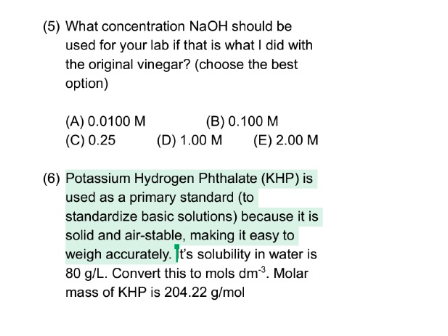I need both of these answered assuming for 5 the volume of NAOH is 100 ml and the actual concentration of acetic acid is 20. (5) What concentration NaOH should be used for your lab if that is what I did with the original vinegar? (choose the best option) (A) 0.0100 M (C) 0.25 (B) 0.100 M (D) 1.00 M (E) 2.00 M (6) Potassium Hydrogen Phthalate (KHP) is used as a primary standard (to standardize basic solutions) because it...

• ### Any help would be great. thank you Calculate the molar concentration of an NaOH solution that...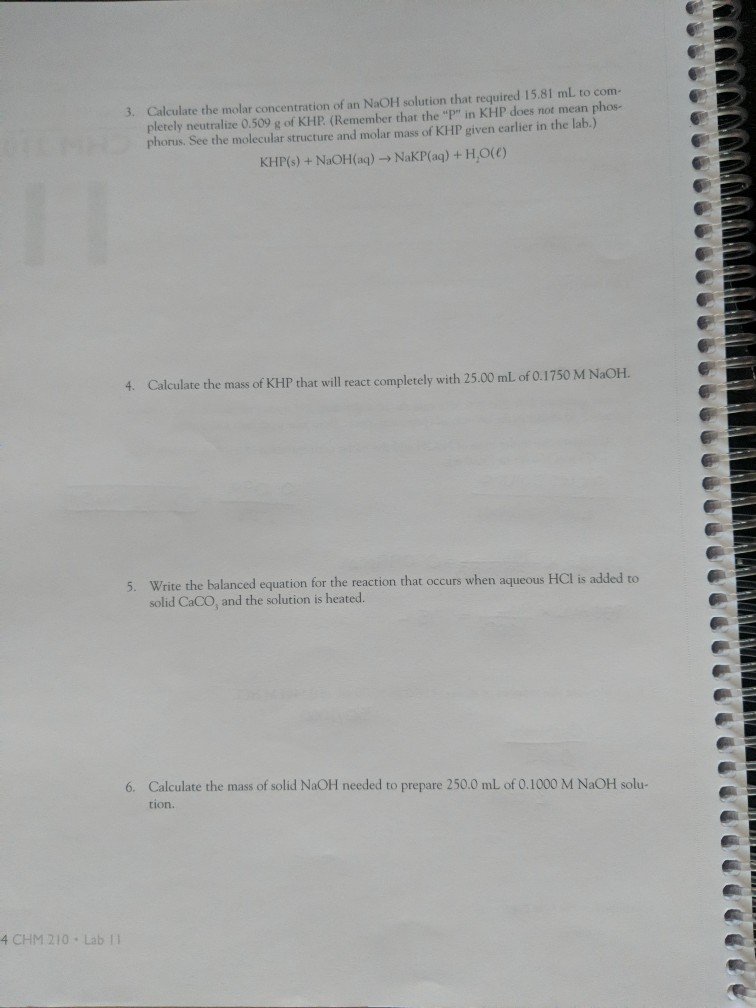Any help would be great. thank you Calculate the molar concentration of an NaOH solution that required 15.81 mL to com- pletely neutralize 0.509 g of KHP (Remember that the "P" in KHP does not mean phos- phorus. See the molecular structure and molar mass of KHP given earlier in the lab.) 3. KHP(s)+NaOH(aq) -NaKP(aq) +H,O(e) Calculate the mass of KHP that will react completely with 25.00 mL of 0.1750 M NaOH 4. 5 Write the balanced equation for the...

• ### (4) If a 1.0L sample of 5% vinegar was diluted with distilled water so that the...(4) If a 1.0L sample of 5% vinegar was diluted with distilled water so that the final volume was 2.4 L what would be the new concentration in mols dm'? (5) What concentration NaOH should be used for your lab if that is what I did with the original vinegar? (choose the best option) (A) 0.0100 M (C) 0.25 (B) 0.100 M (D) 1.00 M (E) 2.00 M (6) Potassium Hydrogen Phthalate (KHP) is used as a primary standard (to...

• ### As part of Lab 11 you will make and standardize a solution of NaOH(aq). Suppose in the lab you measure the solid NaOH an...

As part of Lab 11 you will make and standardize a solution of NaOH(aq). Suppose in the lab you measure the solid NaOH and dissolve it into 100.0 mL of water. You then measure 0.1993 g of KHP (204.22 g/mol) and place it in a clean, dry 100-mL beaker, and then dissolve the KHP in about 25 mL of water and add a couple of drops of phenolphthalein indicator. You titrate this with your NaOH(aq) solution and find that the...

• ### LAB PARTNER Pre-Lab Questions Answer questions 1-6 after you have read the lab experiment and studied...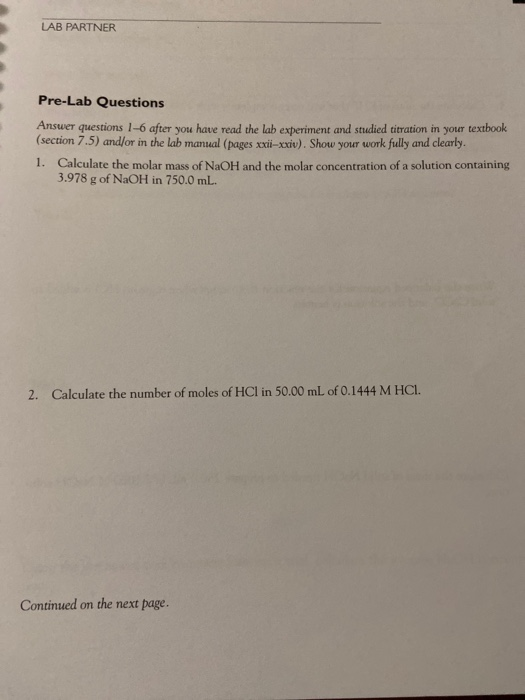LAB PARTNER Pre-Lab Questions Answer questions 1-6 after you have read the lab experiment and studied titration in your textbook (section 7.5) and/or in the lab manual (pages xxi-xxiv). Show your work fully and clearly. Calculate the molar mass of NaOH and the molar concentration of a solution containing 3.978 g of NaOH in 750.0 mL 1. Calculate the number of moles of HCl in 50.00 mL of 0.1444 M HCl. 2. Continued on the next page. 3. Calculate the...

• ### Materials: NaOH MW = 40 g/mL, KHP - potassium hydrogen phthalate, KHC8H404, MW = 204.23 g/mol....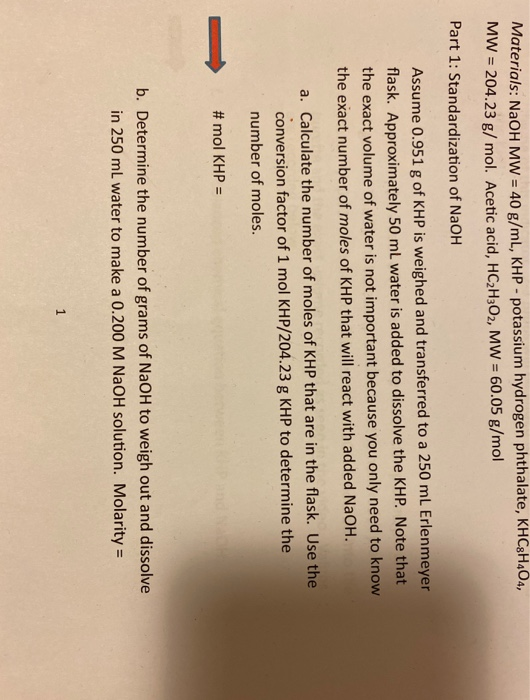Materials: NaOH MW = 40 g/mL, KHP - potassium hydrogen phthalate, KHC8H404, MW = 204.23 g/mol. Acetic acid, HC2H302, MW = 60.05 g/mol Part 1: Standardization of NaOH Assume 0.951 g of KHP is weighed and transferred to a 250 mL Erlenmeyer flask. Approximately 50 ml water is added to dissolve the KHP. Note that the exact volume of water is not important because you only need to know the exact number of moles of KHP that will react with...

• ### I appreciate the help. Thanks you! Week 1 Post-Lab Summary Questions and Activities 1. Why did...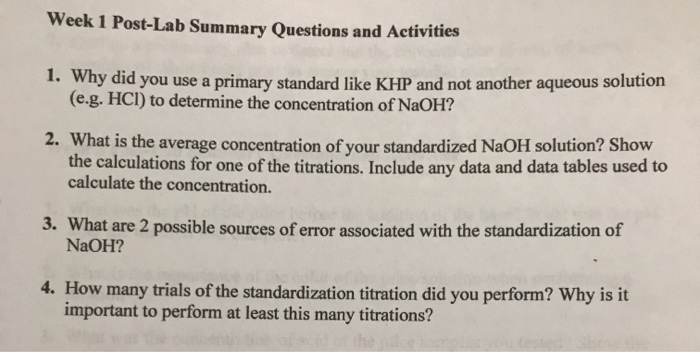I appreciate the help. Thanks you! Week 1 Post-Lab Summary Questions and Activities 1. Why did you use a primary standard like KHP and not another aqueous solution (e.g. HCI) to determine the concentration of NaOH? 2. What is the average concentration of your standardized NaOH solution? Show the calculations for one of the titrations. Include any data and data tables used to calculate the concentration. 3. What are 2 possible sources of error associated with the standardization of NaOH?...